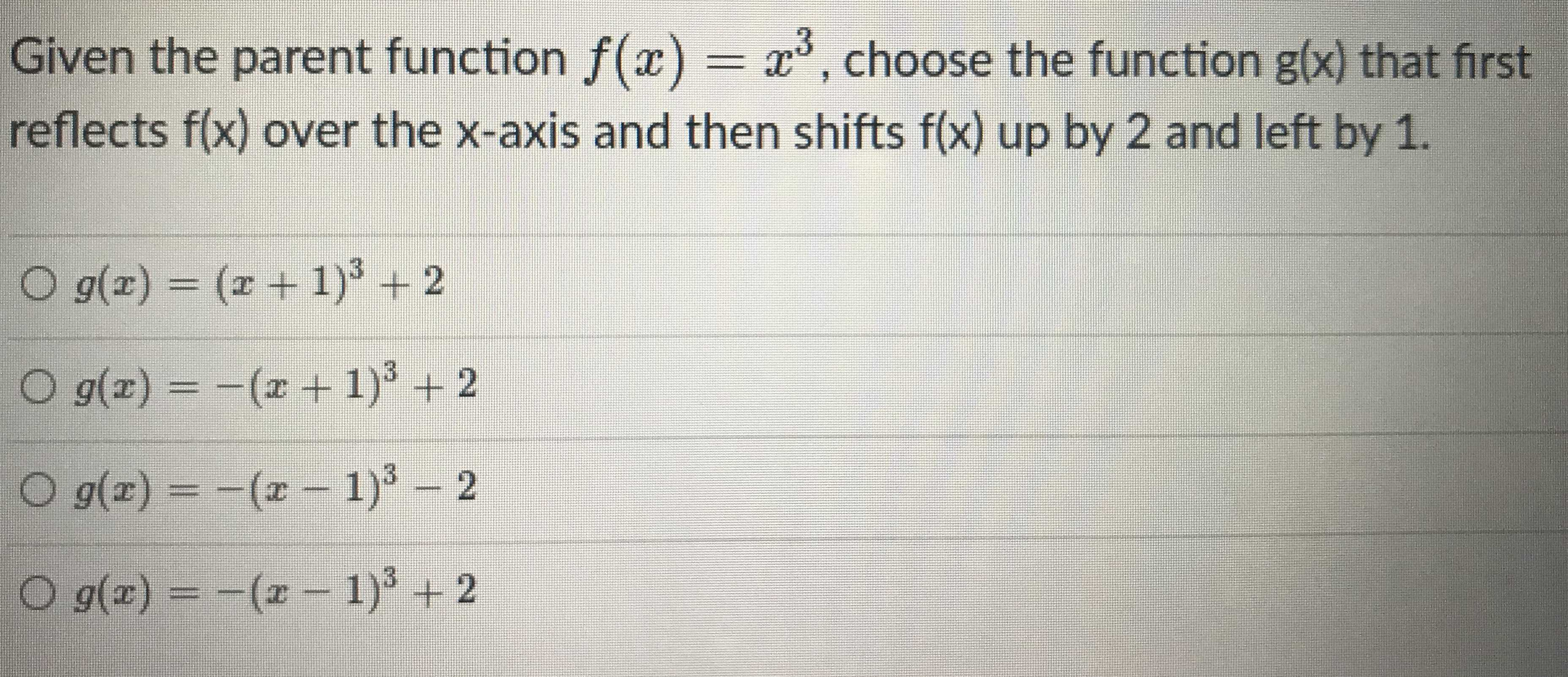### Still have math questions?

Algebra
QuestionGiven the parent function $$f ( x ) = x ^ { 3 }$$ , choose the function $$g ( x )$$ that first reflects $$f ( x )$$ over the $$x$$ -axis and then shifts $$f ( x )$$ up by $$2$$ and left by $$1$$ .

$$g ( x ) = ( x + 1 ) ^ { 3 } + 2$$

$$g ( x ) = - ( x + 1 ) ^ { 3 } + 2$$

$$g ( x ) = - ( x - 1 ) ^ { 3 } - 2$$

$$g ( x ) = - ( x - 1 ) ^ { 3 } + 2$$

$$g(x)= - (x- 1)^{3}+ 2$$